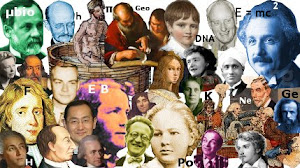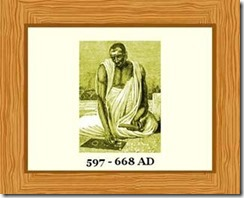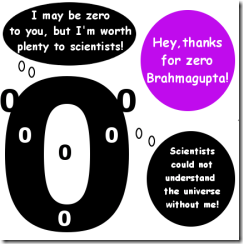### Brahmagupta | Biography of Famous ScientistsBrahmagupta is unique. He is the only scientist we have to thank for discovering precisely zero…Brahmagupta was an Ancient Indian astronomer and mathematician, who lived from 597 AD to 668 AD. He was born in the city of Bhinmal in Northwest India. His father, whose name was Jisnugupta, was an astrologer.
Although Brahmagupta thought of himself as an astronomer who did some mathematics, he is now mainly remembered for his contributions to mathematics.
Many of his important discoveries were written as poetry rather than as mathematical equations! Nevertheless, truth is truth, regardless of how it may be written.

#### Quick Guide to Brahmagupta

##### Brahmagupta:
• was the director of the astronomical observatory of Ujjain, the center of Ancient Indian mathematical astronomy.
• wrote four books about astronomy and mathematics, the most famous of which is Brahma-sphuta-siddhanta ( Brahma’s Correct System of Astronomy, or The Opening of the Universe.)
• wrote that solving mathematical problems was something he did for pleasure.
• was the first person in history to see zero as a number with its own properties.
• defined zero as the number you get when you subtract a number from itself. Identifying zero as a number whose properties needed to be defined was vital for the future of mathematics and science.
• said that zero divided by any other number is zero.
• said that dividing zero by zero produces zero. (Although, this seems reasonable, Brahmagupta actually got this one wrong. Mathematicians have now shown that zero divided by zero is undefined – it has no meaning. There really is no answer to zero divided by zero.)
• was the first person to discover the formula for solving quadratic equations.
• wrote that pi, the ratio of a circle’s circumference to its diameter, could usually be taken to be 3, but if accuracy were needed, then the square-root of 10 (this equals 3.162…) should be used. This is about 0.66 percent higher than the true value of pi.
• indicated that Earth was nearer the moon than the sun
• incorrectly said that Earth did not spin and that Earth did not orbit the sun. This, however, may have been for reasons of self-preservation. Opposing the Brahmins’ religious myths of the time would have been dangerous.
• produced a formula to find the area of any four-sided shape whose corners touch the inside of a circle. This actually simplifies to Heron’s formula for triangles.
• wrote that the length of a year was 365 days 6 hours 12 minutes 9 seconds.
• calculated that Earth was a sphere of circumference around 36,000 km (22,500 miles).
Brahmagupta established rules for working with positive and negative numbers, such as:
• adding two negative numbers together always results in a negative number.
• subtracting a negative number from a positive number is the same as adding the two numbers.
• multiplying two negative numbers together is the same as multiplying two positive numbers.
• dividing a positive number by a negative, or a negative number by a positive results in a negative number.

#### Why is Zero Important?

Although it may seem obvious to us now that zero is a number, and obvious that we can produce it by subtracting a number from itself, and that dividing zero by another non-zero number gives an answer of zero, these results are not actually obvious.
The brilliant mathematicians of Ancient Greece, so far ahead of their time in many ways, had not been able to make this breakthrough. Neither had anyone else, until Brahmagupta came along!It was a huge conceptual leap to see that zero was a number in its own right. Once this leap had been made, mathematics and science could make progress that would have been impossible otherwise.
Brahmagupta might smile at the fact that, without his concept of zero, we would not have the science of thermodynamics; and without thermodynamics we could not even begin to understand the universe – the same universe that Brahmagupta, who viewed himself chiefly as an astronomer, tried so hard to understand over 1300 years ago.